Courses

# Practice Doc: IIFT 2019 For Quant CAT Notes | EduRev

## CAT : Practice Doc: IIFT 2019 For Quant CAT Notes | EduRev

The document Practice Doc: IIFT 2019 For Quant CAT Notes | EduRev is a part of CAT category.
All you need of CAT at this link: CAT

1. You travel by Delhi Metro every day from Botanical Garden, Noida to Hauz Khas, Delhi. At Hauz Khas metro station, you use an escalator to get out of the station. The escalator takes 80 seconds. One day, the escalator was not working and you walk up the escalator in 50 seconds. How many minutes does it approximately take you to walk down the working escalator?
(A) 1.5 minutes
(B) 2.2 minutes
(C) 2.8 minutes
(D) 2.6 minutes

Ans. (B)
Solution:
It is given that by using the escalator, one can get out of the station in 80 sec.
Let us consider the number of steps = 80
Speed of the escalator = 1 step/sec
When the escalator was not working, the time taken by a person = 50 sec
The speed of the person = 80/50 = 8/5 steps/sec
Time taken by a person walking down a moving escalator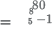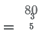= 2.22 min

2. If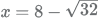andy = 2 + √2, then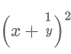is given by:
(A)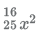(B)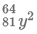(C)(D)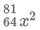Ans.
(D)
Solution: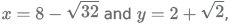We have to find the value of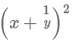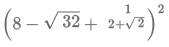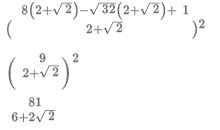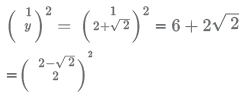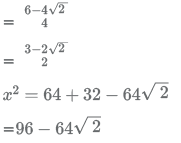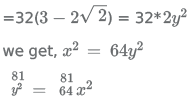3. If the co-ordinates of orthocentre and the centroid of a triangle ABC are (—5, 7) and (5, 5), then the circumcentre of the triangle ABC is :
(A) (25,1)
(B) (10, 4)
(C) (-5,2)
(D) (0,6)

Ans. (B)
Solution:
Centroid divides orthocenter and circumcenter in the ratio of 2:1
The co-ordinates of orthocentre and the centroid of a triangle ABC are (-5, 7) and (5, 5)
Let the co-ordinate of circumcenter be (x,y)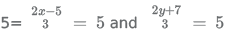x =10 and y = 4
Hence the circumcenter = (10, 4)

4. Consider a cuboidal underground tunnel of length 500 m whose cross-section is given in the figure. If 1m3 of concrete costs 1000 rupees, find the amount of money needed to build the Tunnel.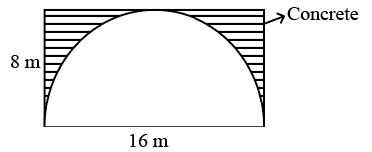(A) 8(4 - π)106 rupees
(B) 64(4 - π)106 rupees
(C) 16(4 - π)10rupees
(D) 32(4 - π)10rupees
Ans. (C)
Solution:
Area of the tunnel to be filled with concrete = 16*8 - π (32)
Length of the length along which the tunnel has to be filled with concrete = 500m
Volume = 16*8 - π (32)*500
= 32(4 - π)*500
Cost of concrete per 1m3 = 1000
Cost of 32(4 - π)*500 = 32(4 - π)*500*1000
= 16(4 - π) * 106

5. In ΔMNL, line NP bisects the angle MNL. If NP : NL = 2: 3 and angle MNL =. Then NP: NL: MN is: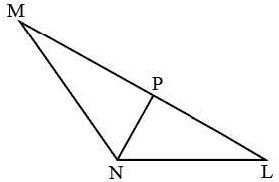(A) 2 : 3 : 4
(B) 2 : 3 : 6
(C) 2 : 3 : 5
(D) 2 : 3 : 9
Ans. (B)
Solution:
Let the value of MN be p
Since NP is the angular bisector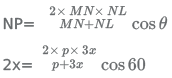p = 6x
NP: NL: MN = 2:3:6

6. A group of women in a society decided to execute interior and exterior decoration of the society in a week’s time. Since 11 women dropped out every day from the second day, the entire decoration was completed on day. How many women participated at the beginning ? (Answer to the nearest integer)
(A) 137
(B) 141
(C) 145
(D) 148
Ans. (C)
Solution:
Let x be the number of women who decided to execute the interior and exterior decoration of the society in a week’s time
Let the efficiency of each of them be 1
Total work = 7x
Number of women who worked on first day = x
Number of women who worked on second day = x-11
Number of women who worked on third day = x-22 ....
On similar lines, the number of women who worked on nth day = x-(n-1)11
Number of women who worked on day 12 = x-121
x+x-11+x-22+........x-(n-1)11 = 7x
12x-(11+22+....121) = 7x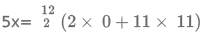x =145

7. Joseph is in a dilemma. He has been offered a job which would pay him ₹ 80,000 per month for first three years and ₹ 1,20,000 per month for the next three years, and ₹ 1,50,000 per month for the remaining four years. He has also been offered an MBA at a prestigious place and he is considering whether to accept the job or go for the MBA. The first year tuition fee for the MBA program is ₹ 16,00,000 and the second year tuition fee for the MBA program is ₹ 20,00,000. After MBA, he'll get a salary of ₹ 2,00,000 per month for the first four years and then ₹ 2,50,000 per month for the remaining four years. What will be the approximate percentage gain for Joseph in opting for the MBA instead of the job in the 10 years horizon considering no discounting of money?
(A) 23%
(B) 25%
(C) 27%
(D) 29%

Ans. (B)
Solution:
The sum accrued by Joseph if he had taken the job = (80000*3+120000*3+150000*4)*12
=144*105
If Joseph has taken the MBA program, tuition fee for the program = 1600000+2000000
Sum accrued post MBA = 200000*12*4+250000*12*4=21600000
Net amount = 21600000-3600000 = 180*105
Net gain if Joseph had taken MBA over job 10 years down the lane= 36*105
Percentage of gain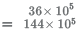= 25%

8. AB is the tangent on the circle at point A. The line BC meets the circle at points C and E. Line AD bisects the angle EAC. If angle EAC 60º = and angle BAC : angle ACB = 2: 5. Find angle ABC: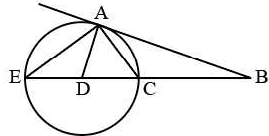(A) 40º
(B) 60º
(C) 30º
(D) None of the options
Ans. (A)
Solution:
Let us consider angle CAB = 2x and angle ACB = 5x
From Apollonius theorem,
angle AEC = 2x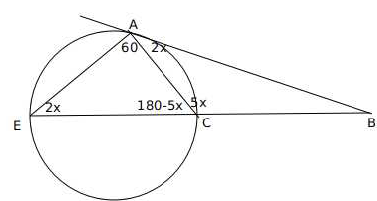In triangle AEC, 60 + 180 - 5x + 2x =180
3x = 60
x = 20
In triangle ABC, angle ABC = 180-7*20 = 40

9. KBC restaurant chain regularly conducts survey of its customers. The customers are asked to rate the food quality, service and price as Excellent, Good and Fair. Customers are also asked whether they would comeback. It was foundthat 76% of customers say that they will come back. Amongst those whosay that they will come back, 57% rate the restaurant as Excellent, 36% rate it as Good and remainderrate it as Fair. Of those whosay that they will not return, the respective values are 14%, 32% and 54%. What percentage of customers rated the restaurant as good?
(A) 27.4%
(B) 35%
(C) 51%
(D) 30.7%

Ans. (B)
Solution:
Let the total customers be 100.
Number of customers who said that they will return = 76x
Number of customers who said that will not return = 24x
Number of customers among those who said that they will return rated the restaurant as good = 76x*0.36
Number of customers among those who said that they will not return rated the restaurant as good = 24x*0.32
Number of customers who rated the restaurant as good = 76x*0.36+24x*0.32 = 35.04x Percentage of customers who rated the restaurant as good = approx 35% B is the correct answer.

10. Four couples are to be seated in a circular table such that each couple sits together. In how many waysthey can sit such that two males sit to the right of their female partners and the other two malessit to the left of their female partners?
(A) 144
(B) 288
(C) 1440
(D) 720
Ans. (B)

11. Nawab has two sons Saif and Amir who have export businesses. Nawab’s satisfaction/ utility level is given by adding twice of the satisfaction level of Saif with the satisfaction level of Amir. If Saif makes a profit of ₹ 100, his satisfaction level goes up by 10% and if he suffers a loss of ₹100, his satisfaction level goes down by 10%. If Amir makes profit of ₹ 100, his satisfaction level goes up by 5% and if he suffers a loss of ₹ 100, his satisfaction level goes down by 15%. Currently, Nawab’s satisfaction level is 24 and the satisfaction level of Saif is the same as the satisfaction level of Amir. If Saif makes a profit of 100 and Amir suffers a loss of ₹ 100, what is the approximate percentage change in Nawab’s satisfaction level?
(A) 1.25%
(B) 1.33%
(C) 1.5%
(D) 1.66%
Ans. (D)
Solution:
Nawab’s satisfaction/ utility level is given by adding twice of the satisfaction level of Saif with the satisfaction level of Amir.
Saif makes a profit of ₹ 100, his satisfaction level goes up by 10% and if he suffers a loss of ₹100, his satisfaction level goes down by 10%.
Amir makes profit of ₹ 100, his satisfaction level goes up by 5% and if he suffers a loss of ₹ 100, his satisfaction level goes down by 15%.
Nawab’s satisfaction level is 24 and the satisfaction level of Saif is the same as the satisfaction level of Amir
Let x be the satisfaction level of Saif
24 = 3x
x = 8
If Saif makes a profit of 100 and Amir suffers a loss of ₹ 100, the satisfaction level of Saif and Amir goes up by 10% and goes down by 15% respectively.
The satisfaction of Saif and Amir = 8.8 and 6.8
Nawab's new satisfaction/ utility level = 2*8.8+6.8 = 24.4
Percentage change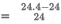= 1.67%

12. A man standing on the line joining the two poles finds that the top of the poles make an angle of elevation of 60º and 45º respectively. After walking for sometime towards the other pole, the angles change to 30º and 60º respectively. The ratio of the height of the poles is:
(A)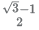(B)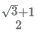(C)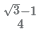(D)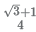Ans. (A)
Solution:
Let 'a' and 'b' be the heights of the two poles
X be the initial position of the man and the angles of elevation be 60º and 45º
Distance between pole 1 and X = a/√3 and distance between pole 2 and X = b
Let Y be the position of the man after walking sometime towards the other pole =
Distance between Y and pole 1 = a/√3 and distance between the pole 2 and Y =  b/√3
Since the distance between the poles will remain the same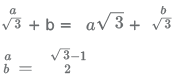13.  An E-rickshaw owner makes 24 trips a month with 4 passengers per trip. If his interest cost for purchase of E-rickshaw is ₹ 120/month, he earns 15 percent profit a month (Profit is the difference between revenue and cost). What will be the approximate percentage profit for the same month if the owner undertakes 20 trips a month with 5 passengers and his interest cost is reduced by 10 percent for the month?
Assume:

(a) Total cost to be proportional to the interest cost.
(b) Revenue per passenger is the same in both cases.

(A) 33.33
(B) 66.67
(C) 72
(D) 100
Ans. A
Solution:
Consider the revenue per passenger = P
⇒ Total revenue = 24*4P = 96P
Since the total cost is proportional to interest, it can be assumed as 120*K
Total cost*(1+Profit/100) = Revenue
⇒ 120K(1+15/100) = 96P ..........(1)
New Revenue = 20*5P = 120*(0.9)K*(1+Profit/100) ..........(2)
Dividing (2) by (1),
25/24 = (0.9/1.15)(1+Profit/100)
Profit/100 = (25*23)/(18*24) - 1 = 1.33-1
⇒ Profit = 33%, Option A is the answer.

14. 400 students were admitted to the 2018-19 MBA batch. 200 of them did not choose “Business Statistics”. 100 of them did not choose “International Management’. There were 80 students who did not choose any of the two subjects. Find the number of students who chose both Business Statistics and International Management.
(A)
180
(B) 220
(C) 280
(D) 300

Ans. (A)
Solution:
The number of students in 2018-19 MBA batch = 400
Number of students who did not choose 'Business Statistics' = 200
Number of students who did not choose 'International Management' = 100
Number of students who did not choose any of the two subjects = 80
Number of students who choose at least one of the two subjects = 400-80=320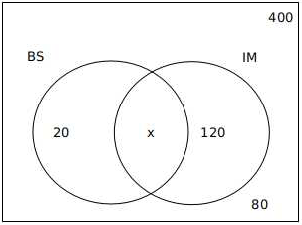140+x = 320
x = 320-140=180

15. At what time between 2.00 pm and 3.00 pm,the two arms of a watch are completely opposite to each other ?
(A) 2.40 pm
(B) 2.44 pm
(C) 2.45 pm
(D) 247 pm
Ans.
(B)
Solution:
Time when the tho hands of the clock are completely opposite to each other between 2.00 pm and 3.00 pm let x be the minutes covered in between 2.00 pm and 3.00 pm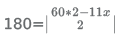x = 43.6 and 21.9

16. The number 37371 - 26371 is divisible by:
(A) 10
(B) 11
(C) 12
(D) 15
Ans. (B)
Solution:
Option A:
37371 − 26371 mod 10
= 7371 − 6371
Since 7 and 10 are co-prime to each other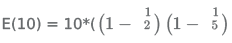= 4
74 mod 10 = 1
74*92 * 73 mod 10 = 1 * 73 mod 10 = 3
6371 mod 10 = 0
Hence 37371 - 26371 is not divisible by 10
Option B:
37371 - 26371 mod 11
4371 - 4371 mod 11
Hence 37371 - 26371 is divisible by 11
Option C:
37371 - 26371 mod 12
1371 - 2371 mod 12
Hence 37371 - 26371 is not divisible by 12
Option D:
37371 - 26371 mod 15
7371 - 11371 mod 15
37371 - 26371 is not divisible by 15

17. A motorboat takes the passengers from Rishikesh to Haridwar and back. Both the cities, Rishikesh and Haridwar are located on the banks of River Ganga. During Kumbh Mela, to earn more money, the owner of the motorboat decided to have more trips from Rishikesh to Haridwar and back, so he increased the speed of the motorboat in still water, by 50%. By increasing the speed, he was able to cut down the travel time from Rishikesh to Haridwarand back, by 60%. Whatis the ratio of the speed of motorboating still water to that of the speed of river Ganga?
(A)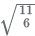(B)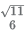(C)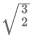(D)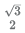Ans. (A)
Solution:
Let the speed of the boat in the still water = 100x and the travel time = 100t and the distance between Rishikesh and Haridwar be 'd'
Let the speed of the river be 100r
Since the owner of the motorboat decides to increase the number of trips, he increased the speed.
Increased speed in still water = 150x
Final travel time = 40t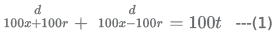After the ease in speed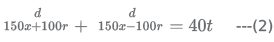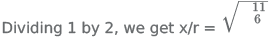18. A cricket team has 11 players and each of them has played 20 matchestill date. Virat, Rohit, Mahendra, Rahul and Shikhar have scored runs at an average of 60, 55, 50, 45 and 40 respectively. Rest of the players have scored at an average of 25 each. In the next 10 matches, Virat and Rohit each scored 900 runs whereas Mahendra scored twice that of Rahul. After 30 matches, if Virat’s new average score is twice that of Rahul, what is the approximate average score of Mahendra?
(A) 49
(B) 41
(C) 43
(D) 45
Ans. (C)
Solution:
The average scores of Virat, Rohit, Mahendra, Rahul and Shikhar in the first 20 matches are 60, 55, 50, 45,40 respectively.
In the next 10 matches, Virat and Rohit each scored 900 runs whereas Mahendra scored twice that of Rahul.
Virat's score in 30 matches = 60*20+900=2100 Let us consider Rahul scored x runs in last 10 matches, then Mahendra scores 2x runs.
Rahul's score in 30 matches = 45*20+x=900+x
Mahendra's score in 30 matches = 50*20+2x = 1000+2x
It is given that
After 30 matches, Virat’s new average score is twice that of Rahul.
2100=2(900+x) x=150
Mahendra's score in 30 matches = 1300
Average score of Mahendra = 43

19. A square of length 1 m is inside a square of length 2 m and four quarter circles are joined as shown in the figure. The value of y - x is given by,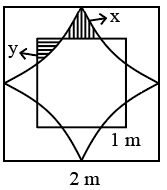(A)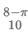(B)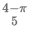(C)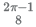(D)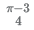Ans. (D)
Solution: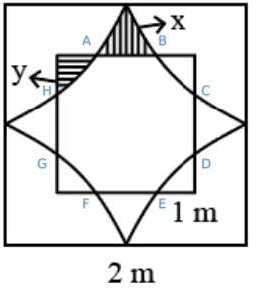From the above figure area of the region bounded by ABCDEFGH = Are of the square with side 2 - (4*quadrants with side 1cm + 4x)
=4-π -4x
Which is same as the area of the square with side 1 cm -4y
4-π -4x = 1-4y
4x-4y=3-π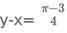20. Rohit purchased a cistern which had a leakage. The cistern can be filled by two inlet pipes, which can individually fill the cistern in 12 min and 15 min respectively. Despite leakage, the two inlet pipes together can fill the cistern in 20 min. How long will it take to empty the completely full the cistern due to leakage?
(A) 10 min.
(B) 12 min.
(C) 15 min.
(D) 16 min.
Ans. (A)
Solution:
Let the capacity of the cistern be LCM of 12 and 15 = 60l
The rate of flow through the inlet pipe 1 = 60/12=5 l/min
The rate of flow through the inlet pipe 2 = 60/15=4 l/min
It is given that despite leakage the two inlet pipes together can fill the cistern in 20 min.
Let the flow through the leakage be x l/min
(5+4-x)20=60
9-x=3
x=6
The leakage will empty the cistern in 60/6=10 min

21. In a triangle, the two longest sides are 13 cm and 12 cm. The anglesof the triangle are in arithmetic progression. The radius ofthe circle inscribed in this triangle is :
(A) √3 cm
(B) √3 - 1 cm
(C) 2 cm
(D) 1 cm
Ans.
(D)
Solution:
Let x be the other side.
It is given that the angles are in AP, so the angles are 60-x, 60, 60+x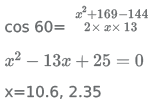Inradii (r) = Area /Semi perimeter
when x = 10.6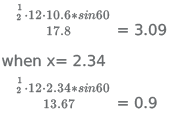x = 1cm(approx)

22. Consider the equation
| x − 5 |2 +5 | x − 5 | −24 = 0
The sum ofall the real roots of the above equationis :
(A) 2
(B) 3
(C) 8
(D) 10
Ans. (D)
Solution:
Let's consider x-5 as 'p'
Case 1: p ≥ 0
| x − 5 |2 +5 | x − 5 | −24 = 0
p2 + 5p − 24 = 0
p2 + 8p − 3p − 24 = 0
p (p + 8) − 3 (p + 8) = 0
(p + 8) (p − 3) = 0
p = -8 and p = 3
x - 5 = 3,x = 8
This is a real root since x is greater than 5.
x - 5 = -8, x = -3.
This root can be negated because x is not greater than 5.
Case 1: p < 0
p2 − 5p − 24 = 0
p2 − 8p + 3p − 24 = 0
p = 8, -3 x - 5 = 8, x = 13.
This root can be negated because x is not less than 5
x - 5 = -3, x = 2. This is a real root because x is less than 5.
The sum of the real roots = 8 + 2 = 10

23. According to birth registration data available with the South Delhi Municipal Corporation,7 babies were born in a particular week in a private hospital. What is the probability that three babies were born on the same day of the week?
(A)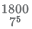(B)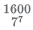(C)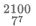(D)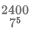Ans. (A)
Solution:
The three babies who are born on the same day of the week can be selected in 7C3 ways
The number of ways in which a particular day can be selected = 7
The remaining four babies can be born in 6*5*4*3 ways
The probability that three babies were born on the same day of the week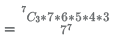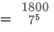24. Monika buys a Samsung’s 360 litre refrigerator from M/s Coldrush Agencies for ₹ 42,000. She makes a down payment of ₹ 12,000 and the remaining amount in 4 equal half yearly instalments. If M/s Coldrush Agencies charge an interest of 10% per annum, approximately what amount Monika has to pay every six month?
(A) ₹ 8230
(B) ₹ 8600
(C) ₹ 8460
(D) ₹ 8620
Ans. (C)
Solution:
Cost of refrigerator = 42,000
Amount paid as downpayment by Monika = 12,000
So the amount on which Monika has to pay the interest = 30,000.
Interest charged per annum = 10%
Since she is repaying the amount half-yearly, the interest = 5%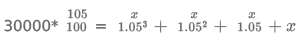31500 = 3.723x
x = 8460

25. Ms. Debjani after her MBA graduation wants to have start-up of her own. For this, she uses ₹ 8,00,000 of her own savings and borrows ₹ 12,00,000 from a public sector bank under MUDRA Scheme.As per the agreement with the bank,she is supposed to repay the principle of this loan equally over the period of the loan which is 25 years. Two years after taking the first loan, she borrowed an additional loan of ₹ 8,00,000 to finance expansion plan of her start-up. If Ms Debjani clears all her loans in 25 years from the date of taking the first loan, how much total interest she has to pay on her initial borrowing? Assume simple interest rate at 8 per cent per annum.
(A) Rs.12,48,000
(B) Rs.12,84,000
(C) Rs.14,20,000
(D) Rs.12,96,000

Ans. (A)
Solution:
For the first year, amount = 1200000*0.08
for the second year = 1152000*0.08
On similar lines for the twenty-fifth year = 48000*0.08
This is in AP with first term 48000*0.08 with common difference 3840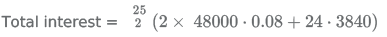= 1248000

Offer running on EduRev: Apply code STAYHOME200 to get INR 200 off on our premium plan EduRev Infinity!

,

,

,

,

,

,

,

,

,

,

,

,

,

,

,

,

,

,

,

,

,

;